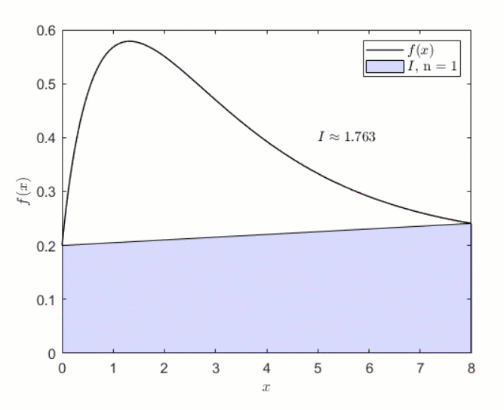Numerical Integration

version 1.0.1 (67.7 KB) by
Interactive courseware module that addresses the fundamentals of numerical integration taught in mathematics courses.

Updated 28 Jun 2021

From GitHub

Numerical IntegrationCurriculum Module created in collaboration with Dr. Matthew Leineweber, San Jose State University.

Created with R2020b. Compatible with R2020b and later releases.Description

This curriculum module contains interactive live scripts that teach two fundamental techniques for approximating definite integrals: the trapezoid and Simpson’s rules. These rules are derived from Lagrange interpolating polynomials and explored through interactive visualizations. Each lesson concludes with a guided activity in which students implement the discussed integration rule. These live scripts can be used as part of a lecture, as activities in an instructional setting, or as an interactive assignment to be completed outside of class.

The instructions inside the live scripts will guide you through the exercises and activities. Get started with each live script by running it one section at a time. To stop running the script or a section midway (for example, when an animation is in progress), use the Stop button in the RUN section of the Live Editor tab in the MATLAB Toolstrip.

Suggested Prework

MATLAB Onramp – a free two-hour introductory tutorial that teaches the essentials of MATLAB.

Details

TrapezoidRule.mlx, TrapezoidRuleSoln.mlx
An interactive lesson that explores the trapezoid rule.

Learning Goals:

• Explain numerical quadrature and its relationship to the definite integral
• Describe how the trapezoid rule is derived
• Illustrate the trapezoid rule graphically
• Compare and contrast integration of a continuous function with integration of tabulated data
• Implement the trapezoid rule in MATLAB

SimpsonsRules.mlx, SimpsonsRulesSoln.mlx
An interactive lesson that explores Simpson’s rules.

Learning Goals:

• Describe how Lagrange interpolating polynomials can be used to derive integration rules
• Illustrate Simpson’s 1/3 rule graphically
• Explain the steps required to implement Simpson’s 1/3 rule
• Compare the accuracy and limitations of Simpson’s 1/3 rule to those of Simpson’s 3/8 rule
• Implement Simpson’s 3/8 rule in MATLAB

Products

MATLAB®

The license for this module is available in the LICENSE.TXT file in this GitHub repository.

Educator Resources

Have any questions or feedback? Contact the MathWorks online teaching team.

Cite As

Brian Hong (2022). Numerical Integration (https://github.com/MathWorks-Teaching-Resources/Numerical-Integration/releases/tag/v1.0.1), GitHub. Retrieved .

MATLAB Release Compatibility
Created with R2020b
Compatible with R2020b and later releases
Platform Compatibility
Windows macOS Linux